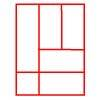#### You may also like### Bracelets

Investigate the different shaped bracelets you could make from 18 different spherical beads. How do they compare if you use 24 beads?### Cut and Make

Cut a square of paper into three pieces as shown. Now,can you use the 3 pieces to make a large triangle, a parallelogram and the square again?### Is a Square a Rectangle?

How many rectangles can you find in this shape? Which ones are differently sized and which are 'similar'?

# Numerically Equal

##### Age 7 to 11Challenge Level

I want to draw a square in which the perimeter is numerically equal to the area.Of course, the perimeter will be measured in units of length, for example, centimetres (cm) while the area will be measured in square units, for example, square centimetres (cm$^2$).

What size square will I need to draw?

What about drawing a rectangle that is twice as long as it is wide which still has a perimeter numerically equal to its area?

Can They Be Equal? offers a suitable extension to this problem.